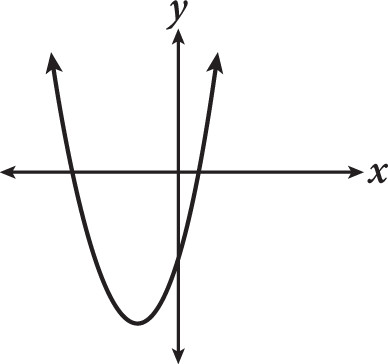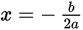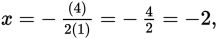# SAT Math Multiple Choice Question 262: Answer and Explanation

### Test Information

Question: 262

7.Which of the following equations could represent the graph in the figure?

• A. y = x2 - 4x - 4
• B. y = x2 + 4x - 4
• C. y = x2 - 8x + 16
• D. y = x2 + 8x + 16

Strategic Advice: Factoring the quadratic equations could give you information about the x-intercepts, but upon inspection, A and (B) can't be factored. As an alternate strategy, find the axis of symmetry using the formula(the quadratic formula without the radical part) to determine in which quadrant the vertex lies. You are looking for an equation whose graph has its vertex in the third quadrant.
Getting to the Answer: Choice (B) is correct becauseand when -2 is substituted into the equation y = (-2)2 + 4(-2) - 4 = -8, it puts the vertex at (-2, -8), which is in the third quadrant and matches the graph.